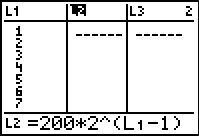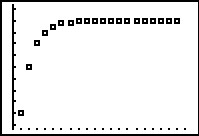••• ##### Device
• TI-83 Plus Family
• TI-84 Plus
• TI-84 Plus Silver Edition
• TI-89 / TI-89 Titanium

# Precalculus: Infinite Geometric Series

by Texas Instruments#### Overview

Students explore infinite geometric series. They will consider the effect of the value for the common ratio and determine whether an infinite geometric series converges or diverges.

#### Key Steps

•In this activity, students will explore infinite geometric series graphically and numerically by changing the common ratio determining for which values the series converges or diverges. Then will then create a scatter plot of the partial sums.

•Students will apply knowledge of the formulas for the sum of finite and infinite geometric series through a medication and drug dosage problem.